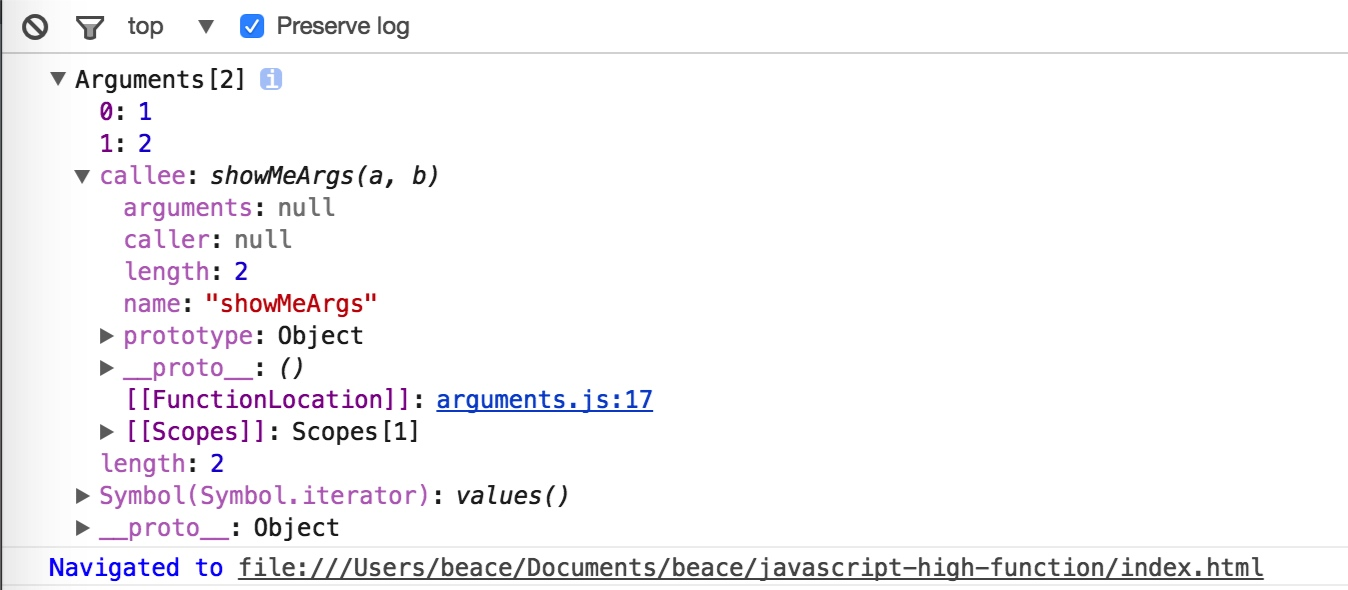### Beace Blog

Written by Beace Lee who lives and works in China building useful things. You should follow him on Twitter

# JavaScript Arguments

March 25, 2017

``````function getArgs(a, b) {
console.log(arguments)
console.log(arguments)
console.log(arguments['0'])
console.log(arguments['1'])
}

getArgs(1, 2);

var b = {"b": "b"};
var b2 = {b: "b"};
console.log(b.b);
console.log(JSON.stringify(b))
console.log(JSON.stringify(b2))``````

``````function getArgs(a, b) {
console.log(arguments.length)
}``````

``````function getArgs(a, b) {
console.log(typeof arguments); // object
}``````

``````const arr = [1,2,3,4];
for (let i = 0; i < arr.length; i ++) {
console.log(a[i]); //1 2 3 4
}``````

``````//将 console.log(a[i]) 替换
console.log(a[i.toString()]) //1 2 3 4``````

``typeof Array //object``

``````var arr = [1,2,3,4,5];
console.log(arr === arr); // true
//这里 arr['01'] === undefined
console.log(arr['01'] === arr['1']) // false``````

``01 === 1 // true``

``'01' === '1' //false``

The 2 in years is coerced into a string by the JavaScript engine through an implicit toString conversion. It is for this reason that ‘2’ and ‘02’ would refer to two different slots on the years object and the following example could be true. —— by Mozilla MDN JavaScript Array

## Arguments0 1 function中的第一个参数
1 2 function中的第二个参数
callee showMeArgs(a, b) 当前执行的函数
length 2 内置属性，获取参数个数
iterator array 返回一个新的Array Iterator对象，该对象包含参数中每个索引的值

### arguments中的参数

#### 特点

• 可以通过arguments的方式获取或设置属性，设置属性时，会覆盖实参
``````function showMeArgs(a, b) {
arguments = 10;
console.log(arguments); //10
console.log(a + b);
}

showMeArgs(1, 2); //12``````
• 当默认参数提供时，实参不会被覆盖
``````function showMeArgs(a = 1, b) {
arguments = 100;
console.log(a + b);
}
showMeArgs(10, 3) // 13``````
• ES6 当使用省略参数（rest parameters）的写法时，arugments也可以返回
``````function showMeArgs(...args) {
console.log(arguments);
}
showMeArgs(1, 2, 3); // object``````

### arguments中的arguments.callee属性

• 先把求和这个动作抽象出来。
``````function plus(n) {
return n === 0 ? 0 : plus(n - 1) + n;
}``````
• 接着迭代数组加求和
``[0,1,2,3,4,5,6].map(n => plus(n)) //[0, 1, 3, 6, 10, 15, 21]``

``[0,1,2,3,4,5,6].map(n => n === 0 ? 0 : (n - 1) + n); // [0, 1, 3, 5, 7, 9, 11]``

``````[0,1,2,3,4,5,6].map(function(n){
return n === 0 ? 0 : arguments.callee(n - 1) + n
});``````

``Uncaught ReferenceError: arguments is not defined``

### arguments个数

``````function getArgs(a, b) {
console.log(arguments.length) //2
}``````

### arguments@@iterator

Array的迭代器。类似Array.prototype.value的使用，现在除Firefox Nightly版本能够体验外，各大浏览器均不支持。例如：

``````var arr = ['w', 'y', 'k', 'o', 'p'];
var iterator = arr.values();
for (let letter of iterator) {
console.log(letter);
}``````

## arguments(object) to array

``````function setColor(color, ele) {
ele.style.color = color;
}``````

``````function setColorReverse(fn) {
return function(color, ele) {
var args = Array.prototype.slice.call(arguments);
fn.apply(this, args.reverse());
}
}
setColorReverse(setColor('container', 'blue'));``````

``[].slice.call(arguments) || Array.from(arguments)``

``````function reverseArgs(fn) {
return function(...args) {
fn.apply(null, [...args].reverse());
}
}

reverseArgs(setColor)(content, 'blue');  // so the color of content is blue``````